##### How to Use FLOOR() Function in PostgreSQL

PostgreSQL provides numerous math functions to perform various mathematical operations, such as ROUND(), SQRT(), etc. All these functions offer different functionality, such as rounding a number to its nearest integer, finding the square root of a number, etc. PostgreSQL offers another useful function named the FLOOR() function that rounds down a number to the next whole number.

This blog post will demonstrate the working of the FLOOR() function via practical examples. So, let’s get started.

How to Use FLOOR() Function in Postgres?

Postgres FLOOR() function is one of the math functions that accept a numeric value or an expression and round down to the next whole number:

`FLOOR(num);`
• The above snippet shows that the FLOOR() function accepts only a single argument, “num”.
• The “num” can be a numeric value or an expression.
• The FLOOR() function retrieves an integer/whole number.
• The return value will always be less than or equal to the given number; the retrieved value cannot exceed the given number.

Example 1: How Does the FLOOR() Function Work?

Let’s execute the below command and see how the FLOOR() function works in Postgres:

`SELECT FLOOR(72.5772);`

In the above snippet, the FLOOR() function takes the number “72.5772”; consequently, it will retrieve the following output: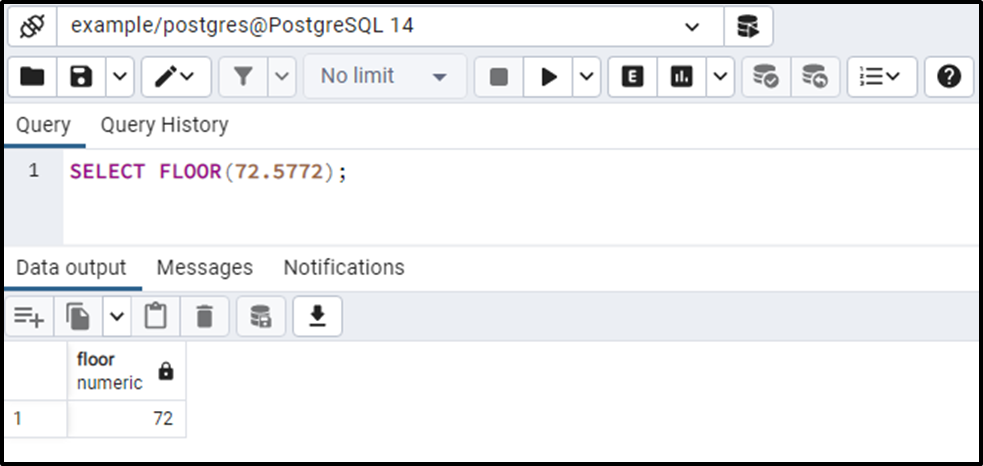The output shows that the FLOOR() function rounded down the given number to the nearest integer.

Example 2: How Does the FLOOR() Function Work With an Expression?

Let’s pass an expression instead of a number and see how the FLOOR() function works:

`SELECT FLOOR(72.5272 + 138.1217);`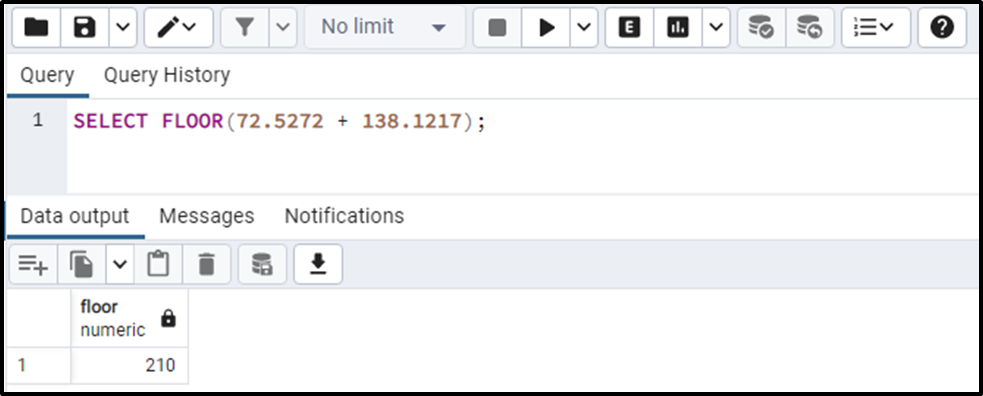The output shows that the FLOOR() function calculates the given expression and rounds the number down to the nearest whole number.

Example 3: How to Use the FLOOR() Function on Table’s Data?

Firstly, we will create a sample table named “emp_bio” with three columns: emp_id, emp_name, and emp_bio:

```CREATE TABLE emp_bio(
emp_id INT,
emp_name TEXT,
emp_sal NUMERIC
);```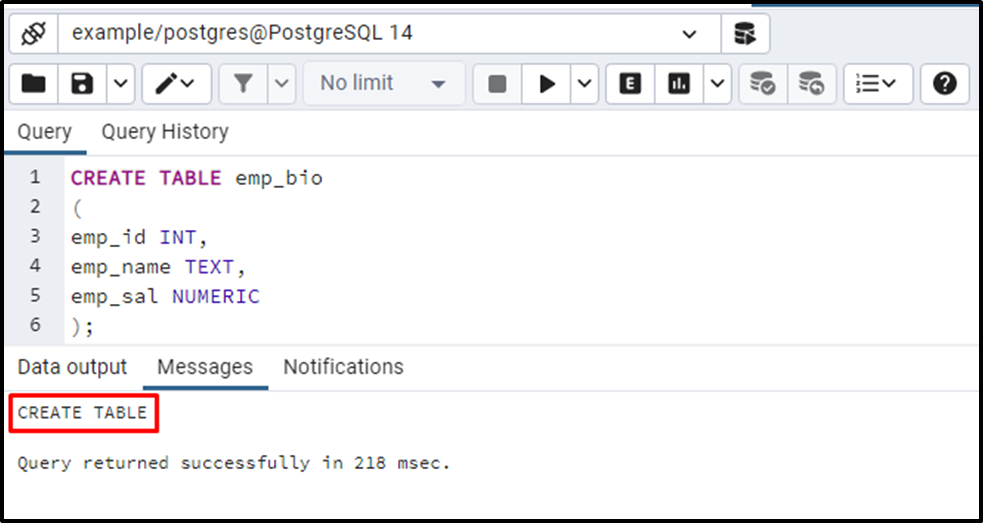The “emp_bio” is successfully created. Now, we will insert some records/data in the newly created table:

```INSERT INTO emp_bio(emp_id, emp_name, emp_sal)
VALUES (1, 'Joseph', 50587.7891),
(2, 'Mike', 40856.5678),
(3, 'Dean', 30756.4321),
(4, 'Ambrose', 30712.1234);```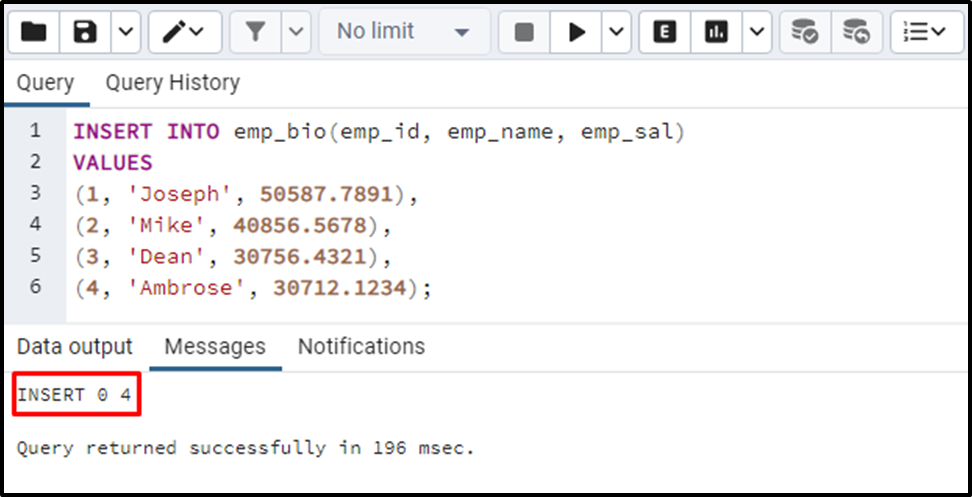Let’s verify the table’s data via the SELECT command:

`SELECT * FROM emp_bio;`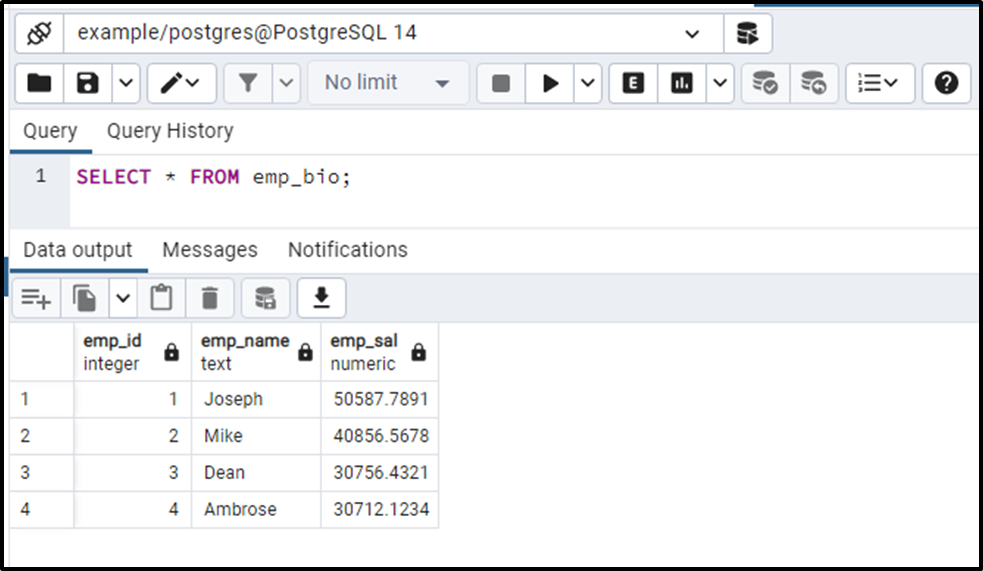Now let’s utilize the FLOOR() function on the emp_sal column of the emp_bio table:

```SELECT emp_sal, FLOOR(emp_sal)
FROM emp_bio;```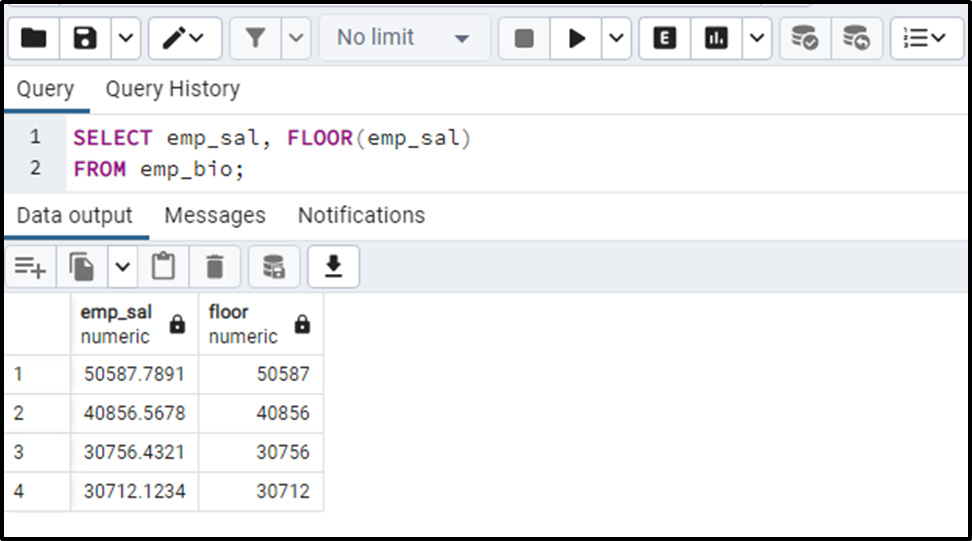The output authenticates the working of the FLOOR() function as it successfully rounded down the column’s values to the nearest integers.

Conclusion

In PostgreSQL, the FLOOR() function is one of the math functions that accept a numeric value or expression and round down the given number to the nearest integer/whole number. The return value will always be less than or equal to the given number; the retrieved value cannot exceed the given number. This blog post presented a detailed guide on the FLOOR() function via practical examples.